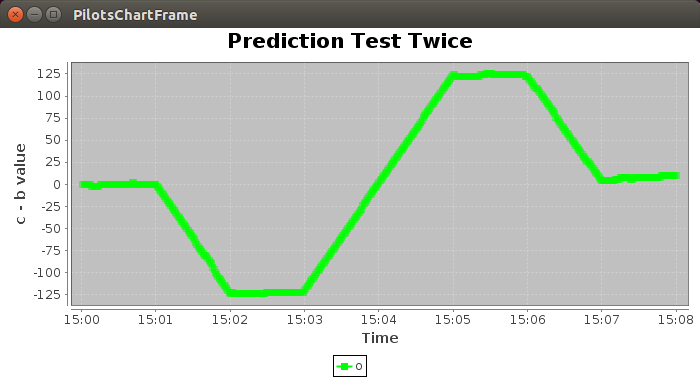# Simple Linear Regression Example

PILOTS home     WCL home
Tutorial index     (Basic ExamplesProgrammingRunningLearning Model OverviewLinear Regression Example)

In this example, a new linear regression learning model will be trained to recognize the relationship b=2a. Then, this learning model will be implemented and the learned relationship will be compared to another output, generated from c.txt, which is similar to b.txt from the Twice (simulated mode) example found from the PILOTS tutorial examples page. As such, this example will produce an output similar to the Twice (simulated mode) example.

# Complete the following steps:

## Build a PILOTS jar file

In `\$PILOTS_HOME`, run the build script:

``````\$ ./build.sh    // (for Windows, use build.bat instead)
``````

After doing so, pilots.jar will be created under the `\$PILOTS_HOME/lib` directory.

## Configure aliases

In order to make sure that aliases for the plc and plcsim compiler commands are correctly figured as well as the definition of \$PILOTS_HOME, make sure that you are in the root directory of the project and then use the following command:

``````source setenv
``````

## Run the learning model server:

In Terminal 1, run server.sh in pilots/util/model

(note: you may need to wait a few seconds for the server to start up)

Make sure that the following data files are added to data/PredictionTest_twice

## Run the Output Handler:

In Terminal 2, make sure to call source setenv from the root directory, and then execute outputHandler in examples/PredictionTest_twice

## Compile and Run the PLT file:

In Terminal 3, make sure to call source setenv from the root directory, and then compile PredictionTest_twice.plt in examples/PredictionTest_twice using the command:

``````plcsim PredictionTest_twice.plt
``````

In Terminal 3, compile the generated PredictionTest_twice.java in examples/PredictionTest_twice

``````javac PredictionTest_twice.java
``````

In Terminal 3, execute the new PredictionTest_twice class file with the appropriate arguments. To simplify this process, execute the script PredictionTest_twice in examples/PredictionTest_twice

(Notice that the classpath is defined within this execution script.)

## Run the Producer:

In Terminal 4, make sure to call source setenv from the root directory, and then (in order to produce the simulation) execute PredictionTest_twiceProducer in examples/PredictionTest_twice

## Output simulated data to the Output Handler:

In Terminal 3, press enter. (After this, some warning messages may appear in this terminal, but they do not hinder the simulation.)

## Final Output

If these steps are executed correctly, you should observe the following output in the Output Handler (terminal 2):Notice that this result is very similar to the Twice (simulated mode) example. In the old example, b was the output of a, and b was explicitly defined as twice that of a. In this learning model example, c was the output of a, and c was learned to be about twice that of a.

Tutorial index     (Basic ExamplesProgrammingRunningLearning Model OverviewLinear Regression ExampleBayes Classifier Example)
PILOTS home     WCL home